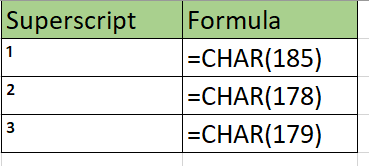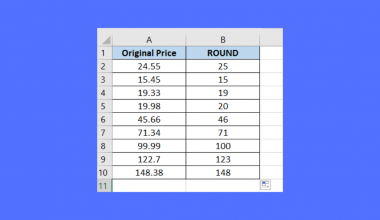# How to Find and Replace Characters with a Superscript in Excel

The Find and Replace function can be used when you need to find and replace a character with a superscript in Excel.

Superscripts are characters set slightly above regular text. They are usually smaller than the rest of the text.

We typically use superscripts in mathematics to denote exponentiation. For example, the equation `x * x * x` can be rewritten as x³.

Since the superscript character is often absent from keyboards, users must rely on either keyboard shortcuts or pasting the character from elsewhere. Keyboard shortcuts for the superscripts of 1, 2, and 3 exist but are often difficult to remember and require a numpad.

For cases where multiple cells need superscripts, it might be difficult to perform these methods for each cell repeatedly. We can use the Find and Replace function to make the changes quickly.

Let’s take a look at a quick example of a sheet where we can use the Find and Replace method.

In the example below, we have strings representing variables with exponents. Each string, however, uses the regular numeric character rather than the superscript character.

With the built-in Find and Replace tool, we can replace all instances of a number with its superscript equivalent in a few clicks.

Now that we know when to use the Find and Replace function to replace a character with a superscript, let’s see how we can make it work on an actual sample spreadsheet.

## A Real Example of Using Find and Replace to Place Superscripts

In this section, we’ll look at a real example of the result of using the Find and Replace tool.

In the example below, the values in column A have been modified by the Find and Replace tool to include the superscript characters ‘²’ and ‘³’.Alternatively, we can use the `SUBSTITUTE` function to replace numbers with their superscript equivalents. We can also use the `CHAR` function to reference a specific superscript. For example, the formula `=CHAR(178)` returns the superscript ‘²’.You can make your own copy of the spreadsheet above using the link attached below.

If you’re ready to try out these methods of adding superscripts to Excel, head over to the next section to learn more.

## How to Find and Replace Character with Superscript in Excel

We will now explain how you can replace characters with superscripts in Excel. You’ll learn how we can use the Find & Replace tool and `SUBSTITUTE` function to add superscripts to your spreadsheets.

Follow these steps to start using the Find and Replace function:

1. First, select the range that contains the values you want to modify with superscripts. In this example, we’ll use the range A2:A8.2. Next, head over to Home > Find & Select > Replace or press the shortcut Ctrl+H.3. In the Find and Replace dialog box, input the string you want to replace in the Find what: input box and add the superscript you want to replace it with in the Replace with: box.4. Click on the Replace All button to perform the replacement. Once the replacement is successful, a dialog box will appear indicating the number of replacements. Click on OK to return to your spreadsheet.5. Repeat the last four steps for each subscript character you want to add. For example, we obtained the result below by replacing both 2 and 3 using the Replace option.We can also use Excel formulas to replace characters with superscripts. We’ll be using the `CHAR` and `SUBSTITUTE` formulas to perform a similar replacement to the previous method. This second process will be useful when processing dynamic data since the formula’s result updates when the original text is updated.

1. If your superscript is 1, 2, or 3, you can use the `CHAR` formulas below to indicate the necessary character to replace.2. To find and replace characters with a superscript, we will use the `SUBSTITUTE` formula. The first argument must refer to the text to change. The second argument will be the character we want to replace, while the third argument will be the superscript we will be replacing that character with.## Frequently Asked Questions (FAQ)

1. What happens when I add a superscript to a number?
Adding a superscript to a number will convert it into a string. For example, a cell with the value ‘2³’ can no longer be considered numeric data if you want to perform exponentiation. You can use the `POWER` function to return a number raised to a given power.

This step-by-step guide should be all you need to find and replace characters with a superscript in Excel. You’ve learned two convenient methods that you can use to add exponents to your mathematical formulas.

The `SUBSTITUTE` function is just one example of a text or string function in Excel that you can use to make your workflow easier. With so many other Excel functions out there, you are guaranteed to find more functions to help improve your worksheets.

Do you want to learn more about what Excel can do? Make sure to subscribe to our newsletter to be the first to know about the latest guides and tutorials from us.### Get emails from us about Excel.

Our goal this year is to create lots of rich, bite-sized tutorials for Excel users like you. If you liked this one, you'd love what we are working on! Readers receive ✨ early access ✨ to new content.

##### You May Also Like## How to Insert Excel Table in Gmail with Borders

Occasionally you’ll want to insert an Excel table directly into your Gmail. Besides the fact that it looks…## How to Remove Decimals with Rounding in Excel

This guide will show and compare several Excel functions that we can use to remove decimals with rounding.…## How to Fix Unable to Move Cells in Excel

This guide will explain how to fix your sheet when you are unable to move cells in Excel.…## How to Set Rounding Precision in Excel

This guide will explain how you can set the rounding precision of numerical values in Excel. Setting the…